## Section29.2Superposition of Forces

The Coulomb's law gives the electric force between two charges. What happens if you have more than two charges?

Experiments show that the force between any two charges is independent of the presence of any other charges. This means that, the force on a charge $q_1$ by two charges $q_2$ and $q_3$ will just be the vector sum of the forces by $q_2$ and $q_3$ given by Coulomb's law for each. This property of the electric force is called the Superposition Principle of Coulomb force.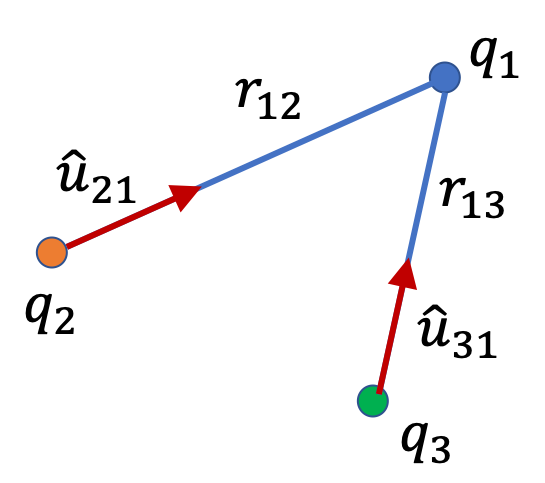\begin{align} \vec F_\text{on 1} \amp = \vec F_\text{on 1}^\text{by 2} + \vec F_\text{on 1}^\text{by 3}, \notag\\ \amp = \dfrac{1}{4\pi\epsilon_0} \dfrac{q_1 q_2}{r_{12}^2} \hat u_{21} + \dfrac{1}{4\pi\epsilon_0} \dfrac{q_1 q_3}{r_{13}^2} \hat u_{31}.\tag{29.2.1} \end{align}

where $\hat u_{21}$ is a unit vector in the direction from 2 to 1 and $\hat u_{21}$ is a unit vector in the direction from 3 to 1.

Alternately, you can work with the components of forces and add them separately to get the components of the force on $q_1\text{.}$

\begin{align} F_\text{on 1,x} \amp = F_\text{on 1,x}^\text{by 2} + F_\text{on 1,x}^\text{by 3}, \tag{29.2.2}\\ F_\text{on 1,y} \amp = F_\text{on 1,y}^\text{by 2} + F_\text{on 1,y}^\text{by 3}, \tag{29.2.3}\\ F_\text{on 1,z} \amp = F_\text{on 1,z}^\text{by 2} + F_\text{on 1,z}^\text{by 3}. \tag{29.2.4} \end{align}

You can then find the magnitude and direction of the force on $q_1$ from these components.

Find the force on the charge at the origin in the following system containing three charges.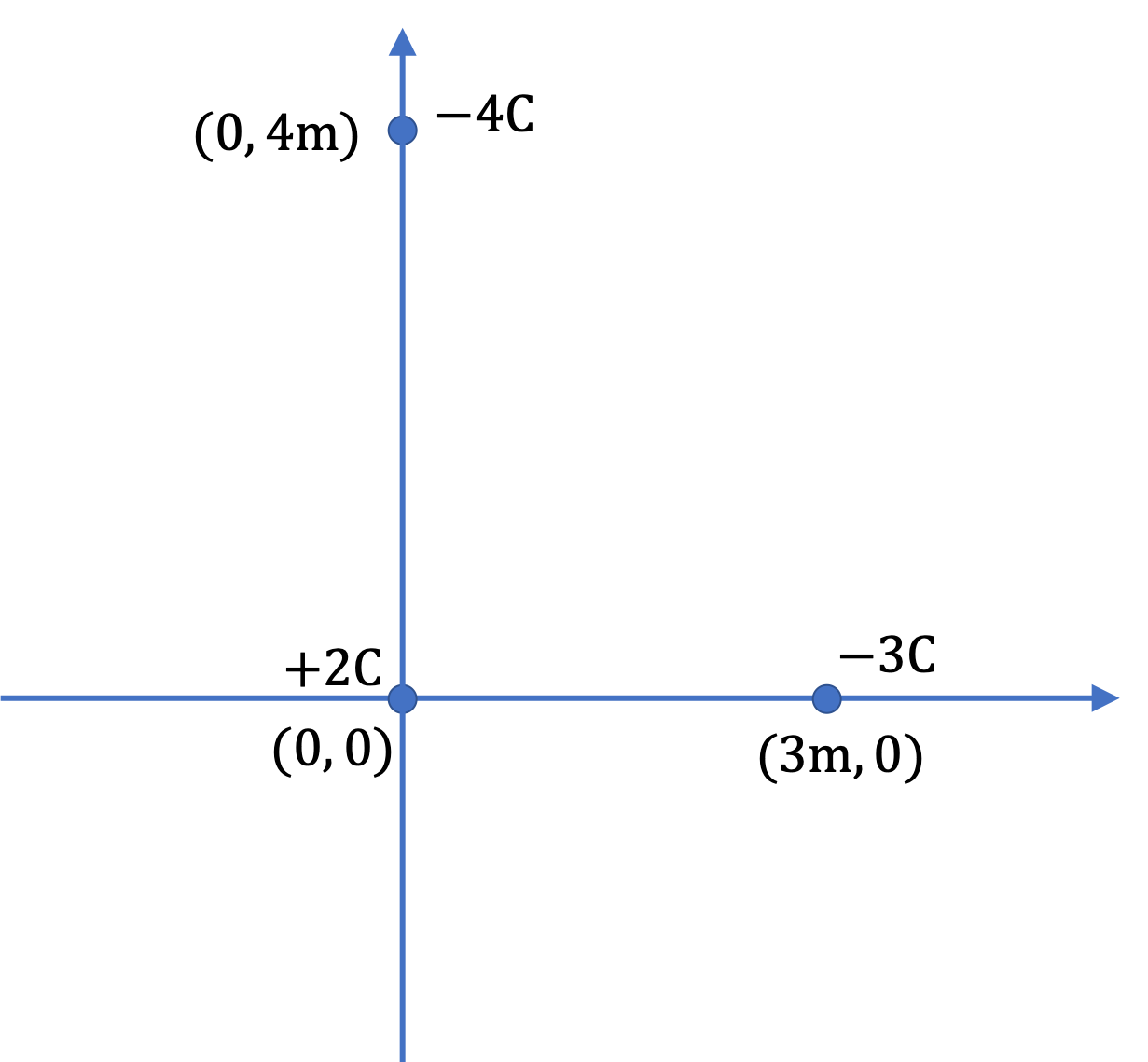Hint

$7.5\times 10^9\text{ N}\text{,}$ $36.9^\circ\text{.}$

Solution

Let's refer to the charge at the origin by subscript 1, the one on the $x$ axis by 2, and the one on the $y$ axis by 3. We need to vectorially sum the two forces $F_2$ and $F_3$ on the charge at the origin to get the magnitude and direction of the Coulomb force on charge 1.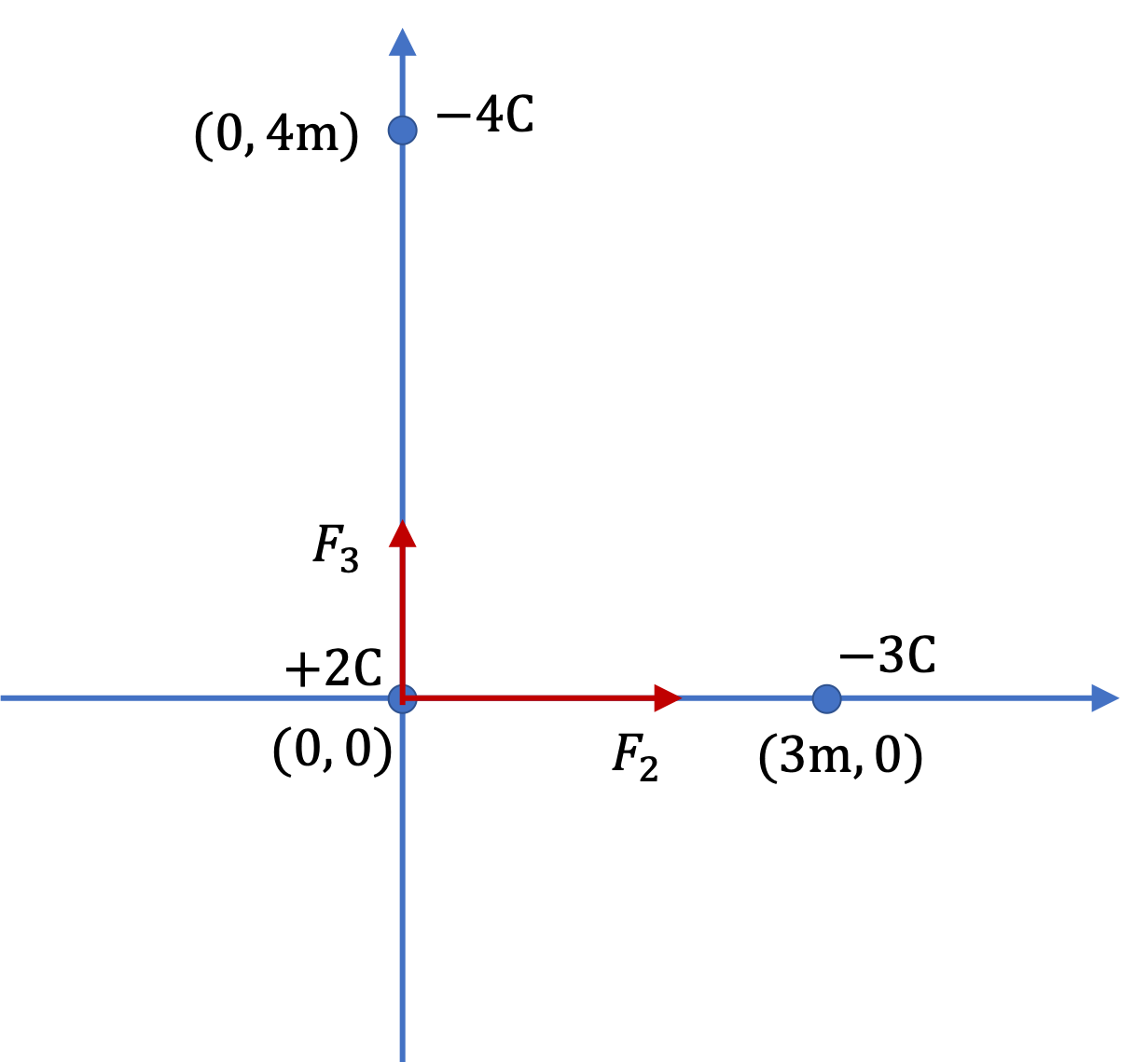First we will work out the magnitudes of the two forces, and then, deduce their Cartesian components, which we will use to find the sum of the vectors. The magnitudes of the two forces are

\begin{align*} F_2 \amp = k\dfrac{q_1 q2}{r_{12}^2} \\ \amp = 9\times 10^{9} \times \dfrac{2\times 3}{3^2} = 6\times 10^{9}\text{ N},\\ F_3 \amp = k\dfrac{q_1 q3}{r_{13}^2} \\ \amp = 9\times 10^{9} \times \dfrac{2\times 4}{4^2} = 4.5\times 10^{9}\text{ N}. \end{align*}

To add these forces, we can't just add the magnitudes. Since they are in perpendicular directions, Pythagora's theorem will give us an easy way to get the magnitude. Let $F$ denote the magnitude of the net force. We can notice that its $x$ and $y$ components are simply

\begin{gather*} F_x = F_{2x}+F_{3x} = 6\times 10^{9}\text{ N}+0,\\ F_y = F_{2y}+F_{3y} = 0+4.5\times 10^{9}\text{ N}. \end{gather*}

Therefore, the magnitude will be

\begin{equation*} F = \sqrt{ F_x^2 + F_y^2 } = \sqrt{ 6^2 + 4.5^2 }\times 10^9 = 7.5\times 10^9\text{ N}. \end{equation*}

and the direction can be given by the counterclockwise angle from the positive $x$ axis since point $(6,\ 4.5)$ is in the first quadrant.

\begin{equation*} \theta = \tan^{-1} \left( \dfrac{4.5}{6} \right) = 36.9^\circ. \end{equation*}

Consider three charges $+2\ \mu\text{C}\text{,}$ $-3\ \mu\text{C}\text{,}$ and $+4\ \mu\text{C}$ held in the $xy$-plane at $(0,0)\text{,}$ $(3\ \text{cm}, 1\ \text{cm})\text{,}$ and $(-2\ \text{cm}, 4\ \text{cm})$ respectively. Find the magnitude and direction of the net Coulomb force on the $+2\ \mu\text{C}$ charge.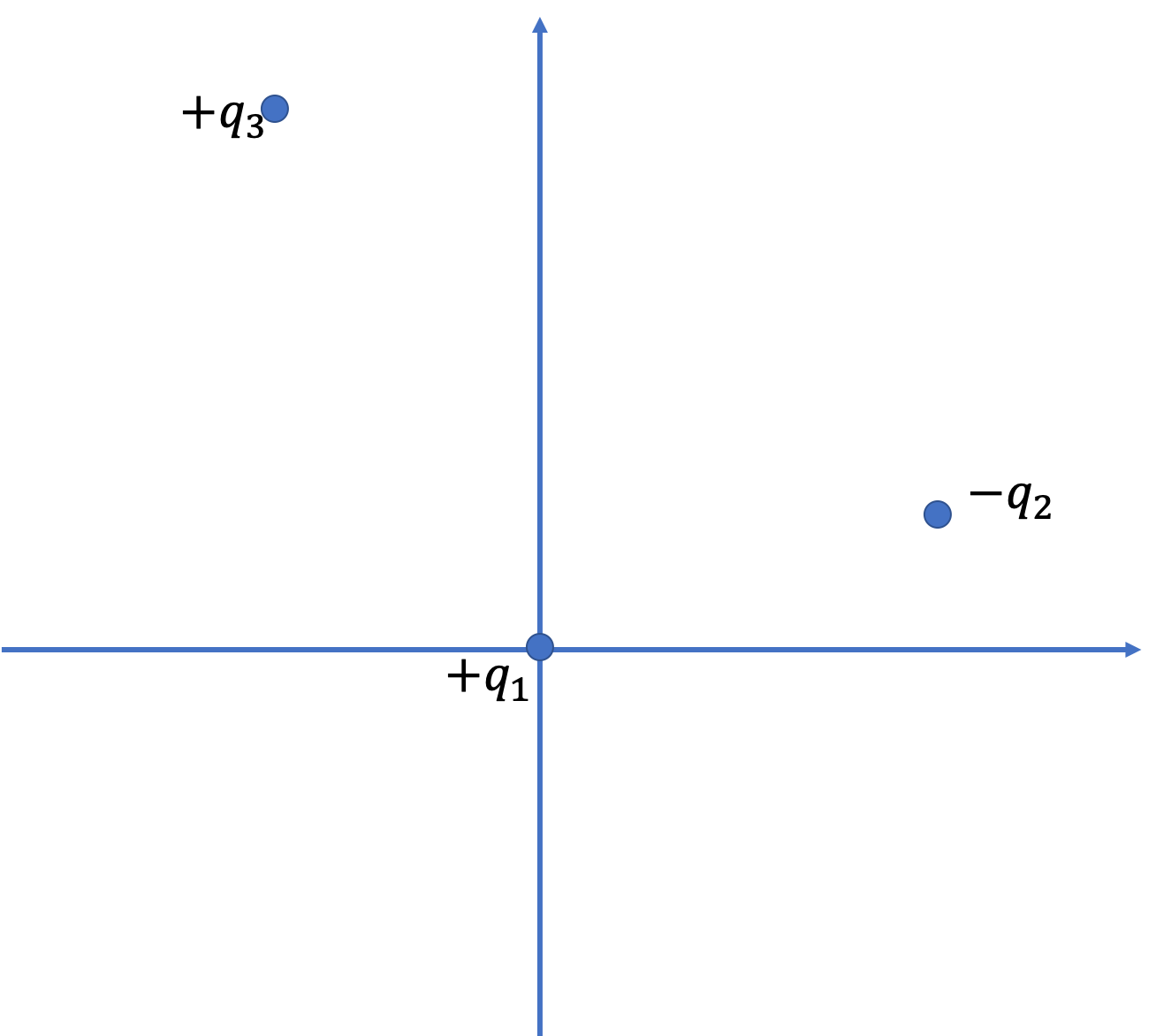Hint

Work with components of the two forces.

$69\text{ N}\text{,}$ $12.7^\circ$ clockwise from positive $x$ axis.

Solution

We will denote $+2\ \mu\text{C}\text{,}$ $-3\ \mu\text{C}\text{,}$ and $+4\ \mu\text{C}$ charges by subscripts 1, 2, and 3 respectively.

First we should work out the direct distances we need for Coulomb's law.

\begin{align*} r_{12} \amp = \sqrt{(x_2-x_1)^2 + (y_2-y_1)^2 } \\ \amp = \sqrt{(3-0)^2 + (1-0)^2 } = \sqrt{10}\text{ cm}. \\ r_{13} \amp = \sqrt{(x_3-x_1)^2 + (y_3-y_1)^2 } \\ \amp = \sqrt{(-2-0)^2 + (4-0)^2 } = \sqrt{20}\text{ cm}. \end{align*}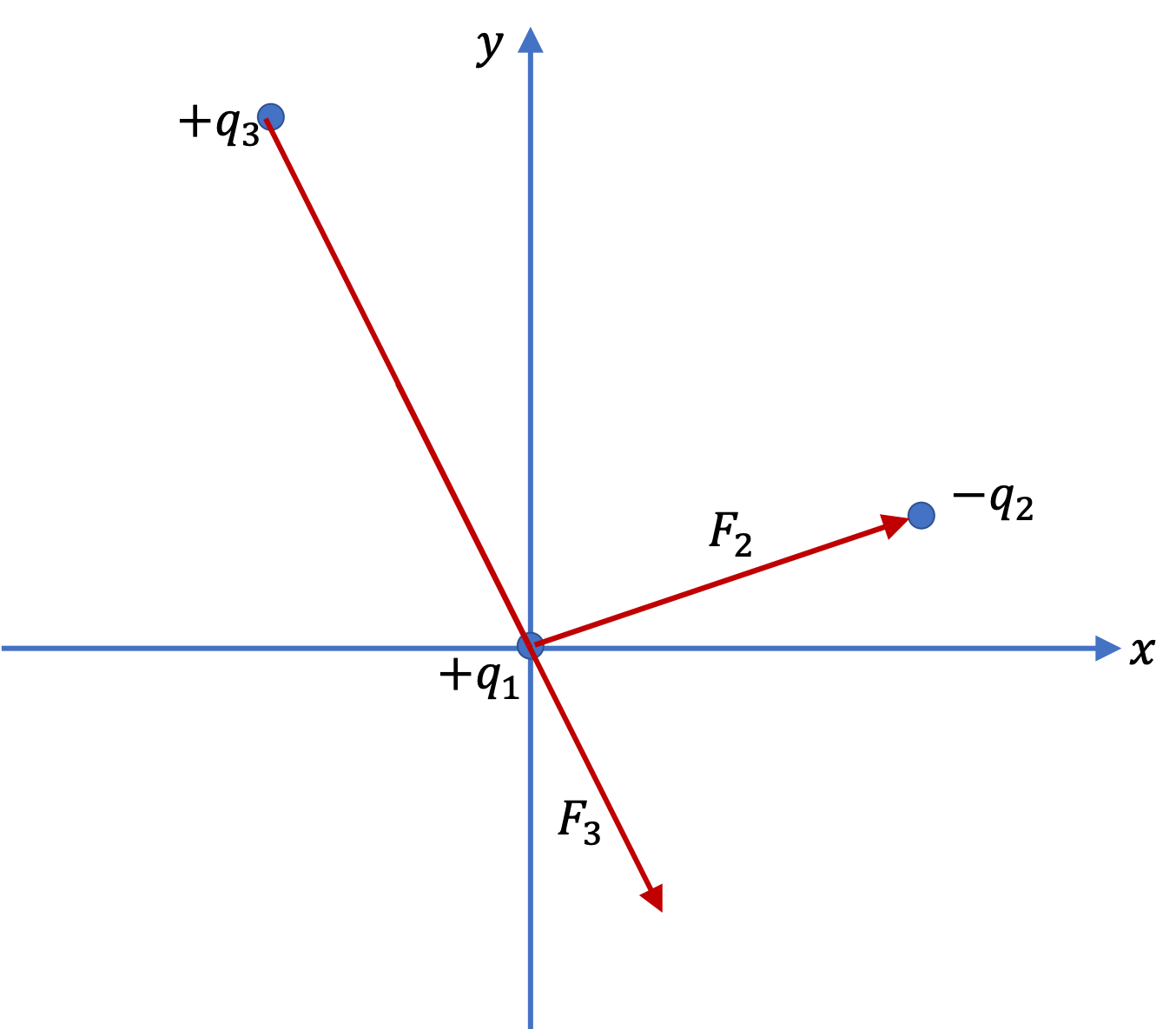Let $F_2$ and $F_3$ be the magnitudes of the Coulomb forces on $q_1$ by the two other charges respectively. The numerical values of these magnitudes are

\begin{align*} F_2 \amp = k\dfrac{q_1 q2}{r_{12}^2}\\ \amp = \left[ 9\times 10^9 \times \dfrac{2 \times 4}{(\sqrt{10})^2} \right]\times \left( 10^{-6}/10^{-2} \right)^2\text{ N},\\ \amp = 54\text{ N}, \end{align*}
\begin{align*} F_3 \amp = k\dfrac{q_1 q3}{r_{13}^2}\\ \amp = \left[ 9\times 10^9 \times \dfrac{2 \times 4}{ (\sqrt{20})^2} \right]\times \left( 10^{-6}/10^{-2} \right)^2\text{ N},\\ \amp = 36\text{ N}, \end{align*}

Sice their directions are not along the same line, we will use $x$ and $y$ components to find their vector sum.

\begin{align*} F_{2x} \amp = F_2\, \dfrac{x_1-x_2}{r_{12}}\times (\text{ sign of }q_1) \times (\text{ sign of }q_2)\\ \amp = 54\text{ N} \times \dfrac{0-3}{\sqrt{10}} \times (+)\times(-) = +51.23\text{ N}. \\ F_{2y} \amp = F_2\, \dfrac{y_1-y_2}{r_{12}}\times (\text{ sign of }q_1) \times (\text{ sign of }q_2)\\ \amp = 54\text{ N} \times \dfrac{0-1}{\sqrt{10}} \times (+)\times(-) = +17.08\text{ N}. \end{align*}

Similarly,

\begin{align*} F_{3x} \amp = F_3\, \dfrac{x_1-x_3}{r_{13}}\times (\text{ sign of }q_1) \times (\text{ sign of }q_3)\\ \amp = 36\text{ N} \times \dfrac{0 + 2}{\sqrt{20}} \times (+)\times(+) = +16.1\text{ N}. \\ F_{3y} \amp = F_3\, \dfrac{y_1 - y_3}{r_{13}}\times (\text{ sign of }q_1) \times (\text{ sign of }q_3)\\ \amp = 36\text{ N} \times \dfrac{0-4}{\sqrt{20}} \times (+)\times(+) = -32.2\text{ N}. \end{align*}

Therefore, the net force $F$ on $q_1$ will have the components

\begin{gather*} F_x = F_{2x} + F_{3x} = 51.23\text{ N} + 16.1\text{ N} = 67.33\text{ N}, \\ F_y = F_{2y} + F_{3y} = 17.08\text{ N} - 32.2\text{ N} = -15.12\text{ N}, \end{gather*}

Thus, the magnitude of this force is

\begin{equation*} F = \sqrt{ F_x^2 + F_y^2 } = \sqrt{67.33^2 + 15.12^2} = 69\text{ N}, \end{equation*}

and the direction will be given by the clockwise angle from the positive $x$ axis since the point $(67.33,\ -15.12)$ is in the fourth quadrant.

\begin{equation*} \theta = \tan^{-1}\left(-15.12/(67.33) \right) = - 12.7^\circ. \end{equation*}

Two charges $+3\ \mu\text{C}$ and $+12\ \mu\text{C}$ are fixed one meter apart. Find the magnitude and direction of the net force on a $-2 \text{ nC}$ charge when it is placed at the following locations:

1. half-way between the two,
2. half a meter to the left of $+3\ \mu\text{C}$ while the other charge is to the right,
3. half a meter above the $+12\ \mu\text{C}$ charge in a direction perpendicular to the line joining the two fixed charges.
Hint

Use vector nature of force when working out the superposition.

(a) magnitude $6.5\times 10^{-4}$ N pointed towards the $\ +12\ \mu$C charge, (b) $3.1 \times 10^{-4}\ \textrm{N}\text{,}$ (c) $8.84\times 10^{-4}\ \textrm{N}\text{,}$ counterclockwise angle $87^\circ$ from negative $x$ axis.

Solution 1 (a)

(a) The forces on the charge $q = -2$ nC are the attractive forces from the two charges $q_1 = +3\ \mu$C and $q_2 = +12\ \mu$C.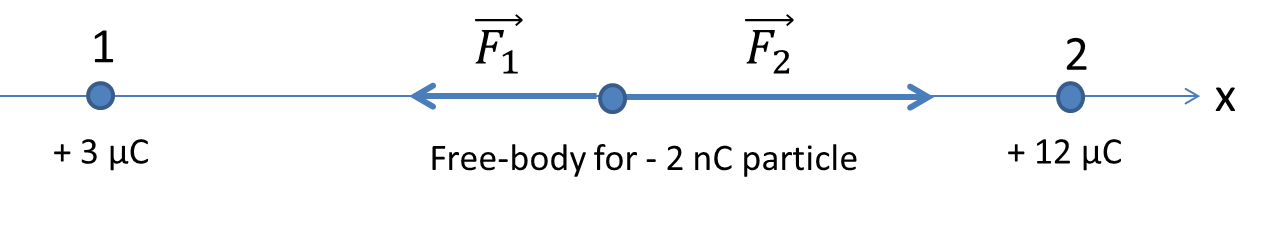Let $r_1$ and $r_2$ be the distances between $q$ and $q_1$ and $q_2$ respectively. Let $\vec F_1$ and $\vec F_2$ be the forces on $q$ by the two charges. If the $x$-axis is pointed in the direction from $q_1$ to $q_2$ then the $x$-component of the net force will be

\begin{equation*} F_x = F_{1x} + F_{2x} = -k\frac{|q_1| |q|}{r_1^2} + k\frac{|q_2| |q|}{r_2^2}. \end{equation*}

The algebra is simplified when we recognize that $q_2 = 4q_1$ and $r_1 = r_2\text{.}$

\begin{equation*} F_x = k\frac{3|q_1| |q|}{r_1^2}. \end{equation*}

Therefore,

\begin{align*} F_x \amp = 9\times 10^9 \ \textrm{N.m}^2/\textrm{C}^2 \times \frac{3\times 3\times 10^{-6}\ \textrm{C} \times 2\times 10^{-9}\ \textrm{C} }{(0.5\ \textrm{m})^2}\\ \amp = 6.5\times 10^{-4} N. \end{align*}

Therefore, the force on the charge $q$ has the magnitude $6.5\times 10^{-4}$ N pointed towards the $\ +12\ \mu$C charge.

Solution 2 (b)

(b) In this part the charge $-2$ nC is placed to the left of $+3\ \mu$C.Again taking the positive $x$-axis pointed to the right we obtain

\begin{equation*} F_x = F_{1x} + F_{2x} = k\frac{|q_1| |q|}{r_1^2} + k\frac{|q_2| |q|}{r_2^2}. \end{equation*}

Now, $r_2 = 3 r_1\text{.}$ Therefore,

\begin{equation*} F_x = k\frac{|q_1| |q|}{r_1^2} \left( 1 + \frac{4}{9} \right) = 3.1 \times 10^{-4}\ \textrm{N}. \end{equation*}
Solution 3 (c)

(c) Now, we have a two-dimensional situation since the forces act in a plane rather than one line.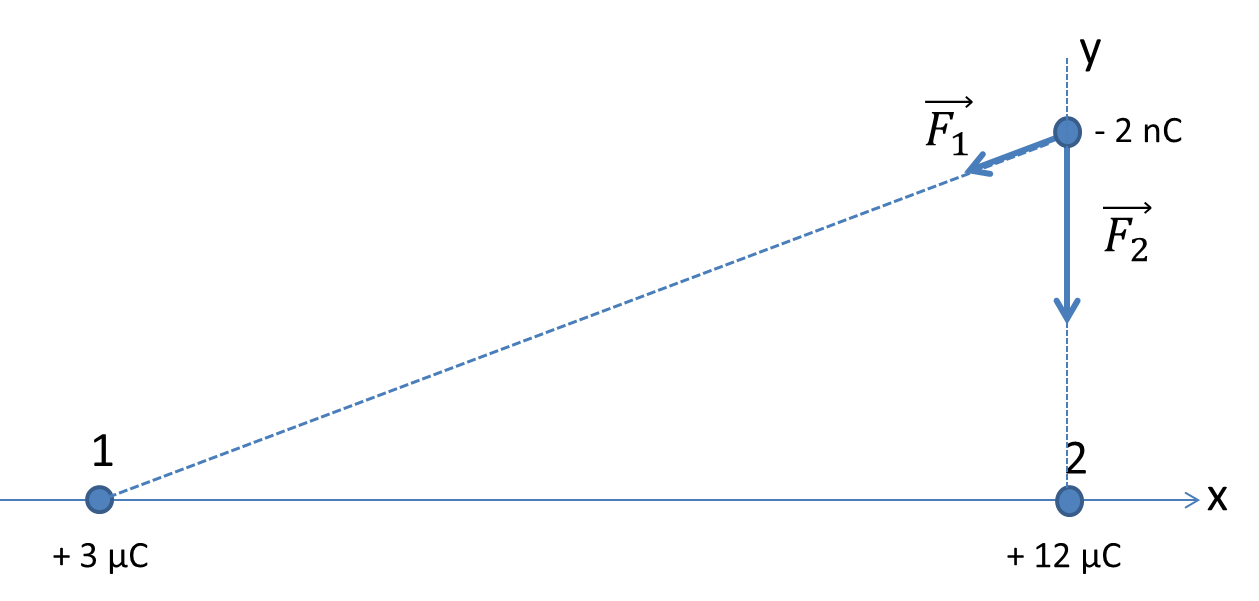To add forces analytically we choose a Cartesian coordinate system with the positive $x$-axis pointed to the right and the positive $y$-axis pointed up. The direct distance $r_1$ and $r_2$ of the charge $q$ from the charges $q_1$ and $q_2$ are

\begin{equation*} r_1 = \sqrt{1^2 + 0.5^2}\ \textrm{m} = 1.118\ \textrm{m},\ \ \textrm{and}\ \ r_2 = 0.5\ \textrm{m}. \end{equation*}

Then the $x$ and $y$-components of the net force will be

\begin{align*} \amp F_x = -k\frac{|q_1| |q|}{r_1^2}\times \left( \frac{1\ \textrm{m}}{1.118\ \textrm{m}} \right)^2 = - 3.86\times 10^{-5} \ \textrm{N}.\\ \amp F_y = -k\frac{|q_1| |q|}{r_1^2}\times \left( \frac{0.5\ \textrm{m}}{1.118\ \textrm{m}} \right)^2 -k\frac{|q_2| |q|}{r_2^2} = - 8.83\times 10^{-4} \ \textrm{N}. \end{align*}

Now, we can compute the magnitude and find the direction of the net force from the $x$- and $y$-components.

\begin{align*} \amp F = \sqrt{F_x^2 + F_y^2} = \sqrt{ 0.386^2 + 8.83^2 }\times 10^{-4} \ \textrm{N} = 8.84\times 10^{-4}\ \textrm{N}.\\ \amp \theta = \tan^{-1}\left(F_y/F_x \right) + 180^{\circ}\ \ [\textrm{since third quadrant}] = 267^{\circ}. \end{align*}

The angle $\theta$ is the counterclockwise angle with respect to the positive $x$-axis. Note the adjustment of $180^{\circ}$ for the direction in the third quadrant.

Four identical charges of magnitude $q$ are located at the corners of a square. The charges are not balanced in force. But if you place appropriate amount of charge $Q$ of the opposite type at the center, you can make all five charges balanced. Find $Q$ in terms of $q\text{.}$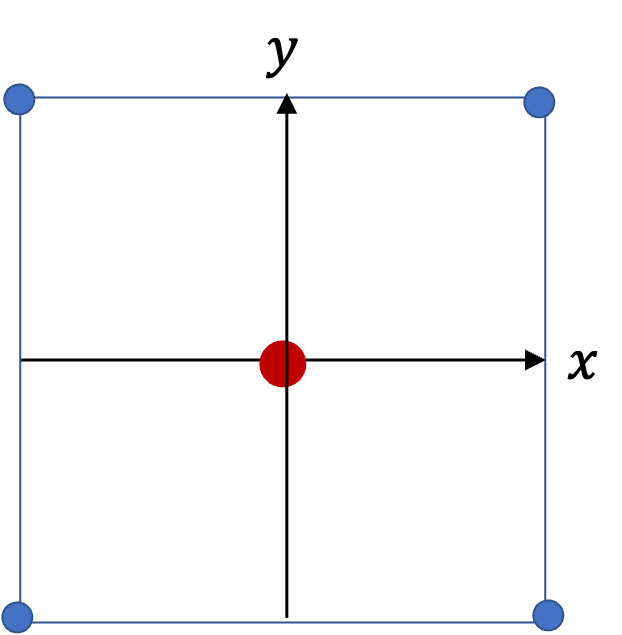Hint

It is sufficient to balance $x$ component on one of the corners. For calculation, assume side of the square is $a\text{.}$

$-\frac{1 + 2\sqrt{2}}{4} \: q \approx -0.957\:q\text{.}$

Solution

From the symmetry, we see that we need to ensure balance of $x$ force on one corner. Let side of the square be $a$ . Working out $x$-component of forces on the charge at the top right corner we get the following condition. Note that $Q$ is negative if we take $q$ as positive.

\begin{equation*} k \frac{q^2}{a^2} + k \frac{q^2}{ (a\sqrt{2})^2 } \frac{1}{\sqrt{2}} + k \frac{q Q}{ ((a/2)\sqrt{2})^2 } \frac{1}{\sqrt{2}} = 0. \end{equation*}

Canceling out common factors and solving for $Q\text{,}$ we get

\begin{equation*} Q = - \frac{1 + 2\sqrt{2}}{4} \: q \approx - 0.957\:q. \end{equation*}

Six identical charges of magnitude $q$ are located at the corners of a regular hexagon. The charges are not balanced in force. But if you place appropriate amount of charge $Q$ of the opposite type at the center, you can make all seven charges balanced. Find $Q$ in terms of $q\text{.}$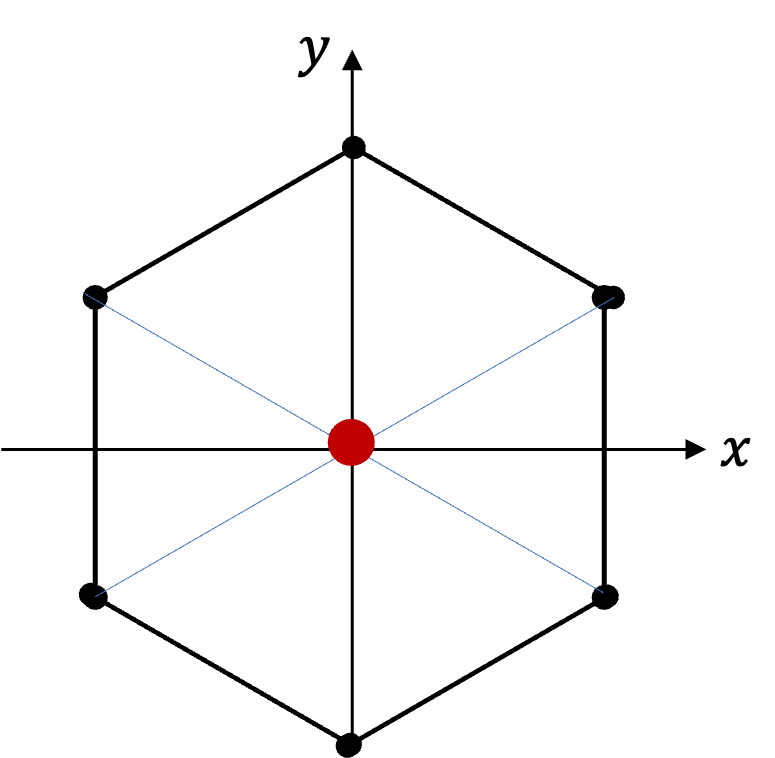Hint

It is sufficient to balance $x$ component on one of the corners.

$-\frac{45 + 4\sqrt{3}}{36} \: q \approx -1.44\:q\text{.}$
From the symmetry, we see that we need to ensure balance of $x$ force on one corner. Let side of the hexagon be $a\text{.}$ Working out $x$-component of forces on the charge at the diagonal top right corner we get the following condition. I went around the hexagon in counterclockwise and then the center and found the direct distance and the angle for $\cos$ that will give the $x$ component of the force. Note that $Q$ is negative if we take $q$ as positive.
Canceling out common factors and solving for $Q\text{,}$ we get